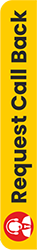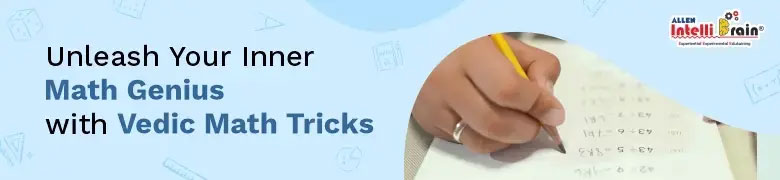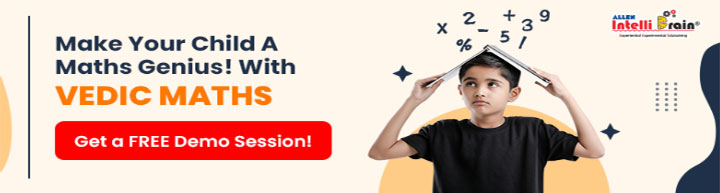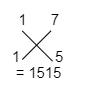# Vedic Maths Tricks For Faster Calculations

June 02, 2023The Sanskrit word "Veda'' means "Knowledge" in English. This Sanskrit word is where the phrase Vedic Mathematics originates. Vedic Maths is a century-old mathematical approach that consists of methods or techniques for solving mathematical problems efficiently and accurately.

Indian mathematician Jagadguru Shri Bharathi Krishna Tirthaji, who is known as the father of Vedic mathematics, made the discovery between 1911 and 1918.

The term Vedic Maths Tricks refers to the group of techniques or methods in vedic mathematics. Numerous mathematical problems, including those involving algebra, geometry, trigonometry, conics, and more, can be solved with the help of Vedic Maths Tricks. With the use of sutras or formulae, vedic mathematics tricks can be performed. These sutras are further divided into 16 Sutras (Formulae) and 13 Sub-Sutras (Sub Formulae).

## Best Vedic Maths Tricks for Addition, Multiplication, Subtraction & Division

Here are 8 Vedic Maths Tricks for Fast Calculations.

### Trick 1: Vedic Mathematics tricks for Squaring a number with unit digit 5

Step 1: 95 x 95 = ___25 (the answer will always end with 25)

Step 2: Now, multiply the first digit of the

two-digit number by (the first digit+1).

That is, 9 * (9+1) = 9 * 10

= 90

So the answer is, (95) ² =9025.### Trick 2: Vedic Maths tricks for subtraction

Subtracting any number from 1000, 10000, 100000 and so on, For example:- 10000 - 7478 = ?

Except for the last digit, subtract each of the

digits in 7478 from 9. The last digit (8) must be subtracted

from 10.

Step 1: 9 - 7 = 2 (First digit of the answer)

Step 2: 9 - 4 = 5 (Second digit of the answer)

Step 3: 9 - 7 = 2 (Third digit of the answer)

Step 4: 10 - 8 = 2 (Last digit of the answer)

So the answer is, 10000 – 7478 = 2522.

### Trick 3: Vedic Maths Multiplication tricks

Multiplying any number by 11, For example:- 63 * 11

Step 1: Write “0” in the beginning at the end of any number

= 0630

Step 3: Now add in pair of two from left to right

= (0+6)/(6+3)/(3+0) = 693

So, the answer is, 63 * 11 = 693.

### Adding a two-digit number, For example:- 38 + 97 =?

Step 1: Find out the number which is closest to the 10s multiple

The closest to the 10s multiple for 38 is 40

The closest to the 10s multiple for 97 is 100

Step 2: Add the numbers which are the multiples of 10s

40+100 = 140.

Step 3: Add/Subtract the deficiency of numbers.

The deficiency of number 38 is 2

The deficiency of number 97 is 3

So, 140 – (2 + 3) = 140 – 5

= 135

### Trick 5: Vedic Maths Multiplication tricks

Multiplying any number with 5, For example: 6284 x 5=?

Step 1: Divide the number 6284 by 2.

6284/ 2 = 3142

Step 2: The trick here is to add 0 at the end of the number if the result above is a whole number, and 5 at the end if it is not, and ignore any remainder you have.

Since 3142 is a whole number, so add 0.

That would be 31420.

So, the answer is, 6284 x 5= 31420

### Trick 6: Vedic Maths tricks for division

Dividing a Large number by 5, For example: 3456 / 5 = ?

Step 1: Multiply the number by 2.

3456 * 2 = 6912

Step 2. Move one decimal place.

That would be, 691.2

So, the answer is 3456 / 5 = 691.2

### Trick 7: Vedic Maths Multiplication tricks

Multiplying any two - digit numbers, For example: 17 * 15 = ?

Step 1. Multiply the unit digits of both numbers.

17 * 5 = 35.

Step 2. Keep the unit digit of 35 in the unit's place & write ‘3’ as carry.

Step 3. Multiply the digits crosswise of both the 2-digit numbers & add the product & also add the carry ‘3’.Step 4. Keep the unit digit & carry forward ‘5’ & carry forward ‘1’ in the next part

1 | 12 | 35
= 255

So, the answer is = 255.### Trick 8: Vedic Maths Multiplication tricks

Multiplying any three - digit numbers, For example: 104 * 108= ?

Step 1: find the deviation of the given numbers

104 = 4

108 = 8

Step 2: Choose any number and add the deviation the other number

108 + 4 = 112

Step 3: Now multiply the deviations

4 X 8 = 32

Step 4. Now combine both parts.

104 + 4
108 + 8
= 11232

It is fairly evident from the Vedic Maths Tricks mentioned above how simple some mathematical problems are to solve. Enroll now in ALLEN IntelliBrain Vedic Maths Courses for Kids of grade 1 to 8 (Above)

## Benefits Of Learning Vedic Mathematics Tricks for Kids

Some of the benefits of learning Vedic Maths tricks are as follows:

• It speeds up the calculation of Mathematical problems by 10-15 times when compared to traditional Maths procedures. Thus, giving a greater advantage to kids when they are attending any competitive exams.
• It promotes a holistic approach to learning and alleviates the burden of remembering difficult Mathematical concepts. As a result, the kid is relaxed and more confident while facing any mathematical questions.
• It helps in accurately solving Mathematical problems and the reduction of silly mistakes.
• It also improves concentration, memory, IQ, and the ability to make wise decisions.
• Vedic Maths enhances the left and right sides of the brain by enhancing visualisation and concentration abilities, according to research. Additionally, it enhances mental clarity and intellect.
• It reduces Maths stress and, more importantly, removes Maths phobia in kids.

Given the advantages listed above, it is clear why it is crucial to educate children Vedic Mathematics. There are several online and offline vedic lessons accessible for children. For kids in grades 1 to 8 (and above), one such online course is ALLEN Intellibrain Vedic Maths classes.

The early childhood development team created the course outline, and highly qualified specialists are in charge of instructing it. The child's speed, accuracy, and conceptual grasp are regularly assessed via Live Assessments, and parents also receive a thorough Student Performance Report.

The child receives a certificate of completion at the conclusion of each level. This gives the child an even greater sense of success. These programmes have earned top rankings among vedic mathematics classes for kids in India thanks to the excellent praises from both parents and students.